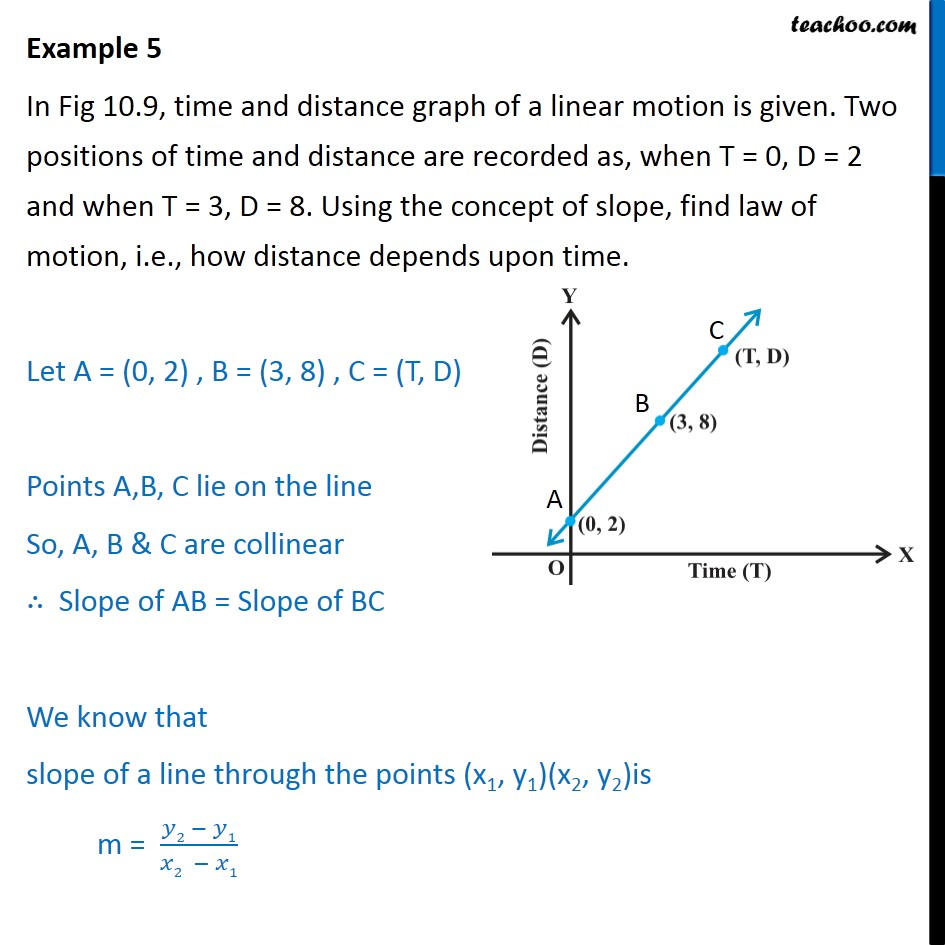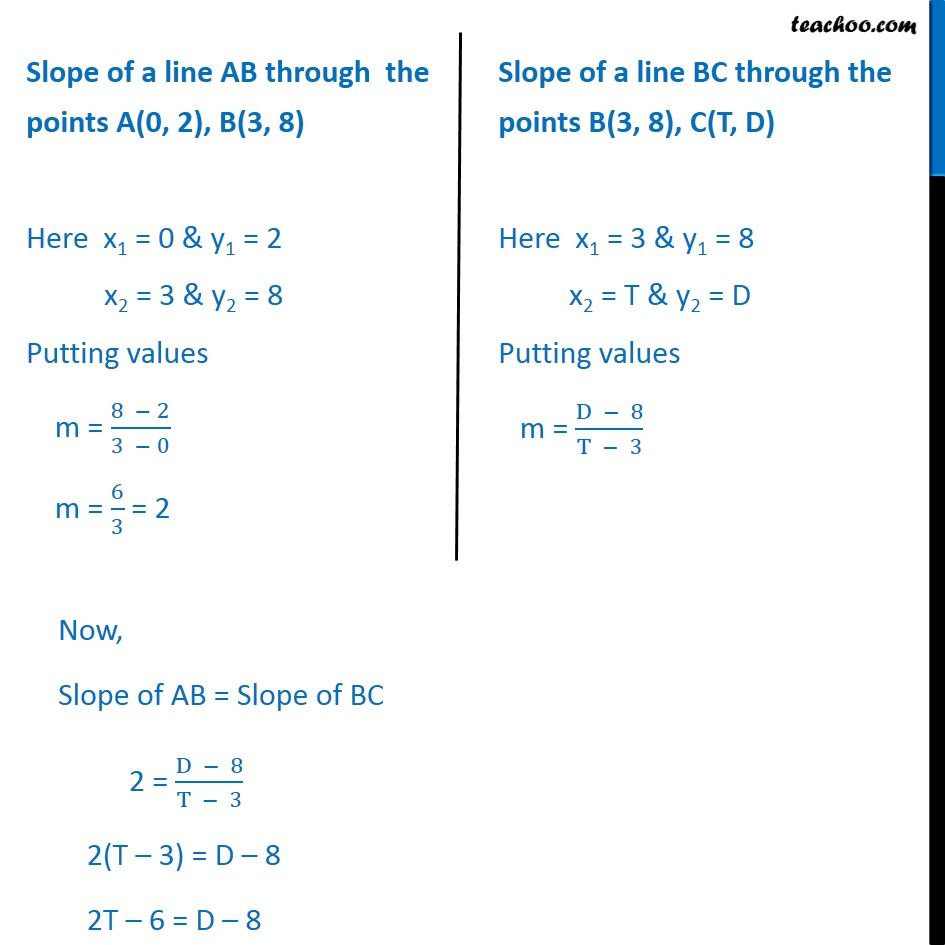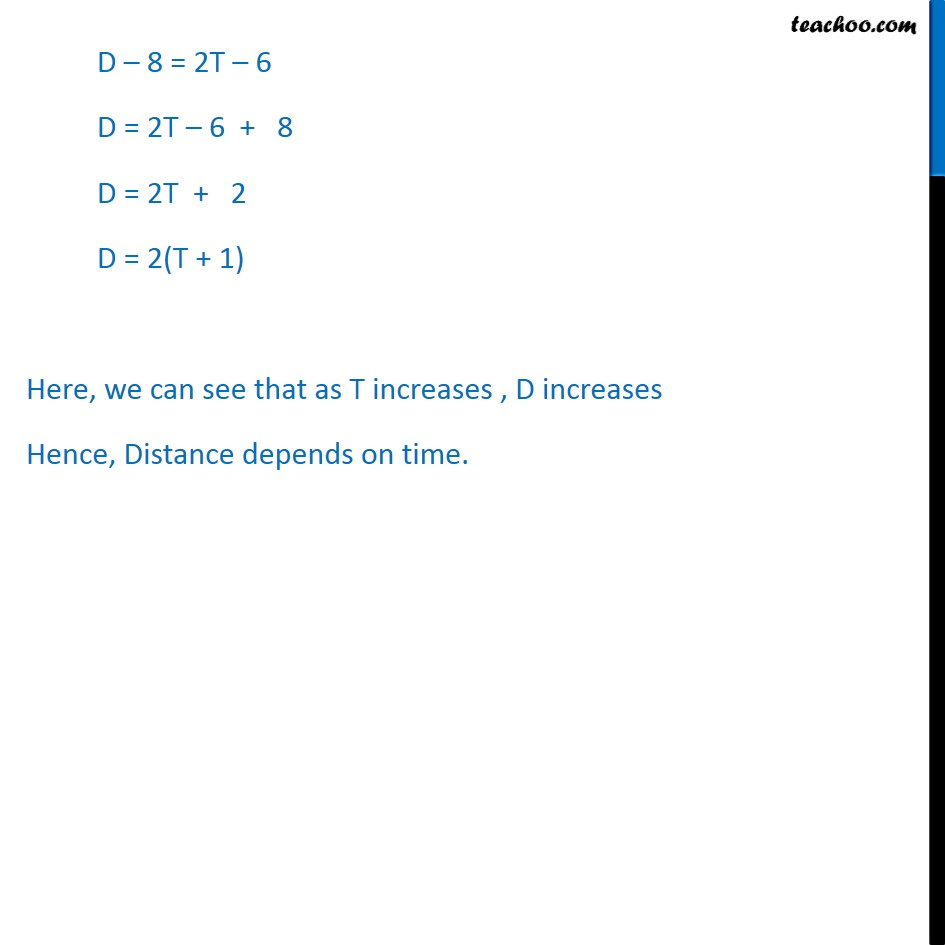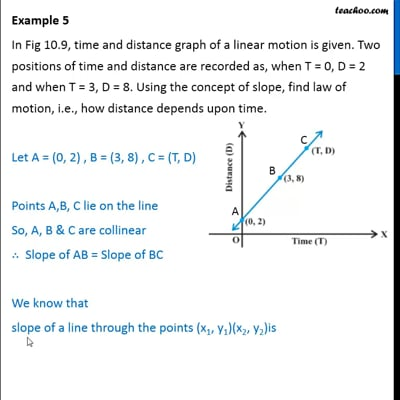Examples

Chapter 10 Class 11 Straight Lines (Term 1)
Serial order wiseThis video is only available for Teachoo black users

### Transcript

Example 5 In Fig 10.9, time and distance graph of a linear motion is given. Two positions of time and distance are recorded as, when T = 0, D = 2 and when T = 3, D = 8. Using the concept of slope, find law of motion, i.e., how distance depends upon time. Let A = (0, 2) , B = (3, 8) , C = (T, D) Points A,B, C lie on the line So, A, B & C are collinear Slope of AB = Slope of BC We know that slope of a line through the points (x1, y1)(x2, y2)is m = ( 2 1)/( 2 1) D 8 = 2T 6 D = 2T 6 + 8 D = 2T + 2 D = 2(T + 1) Here, we can see that as T increases , D increases Hence, Distance depends on time.